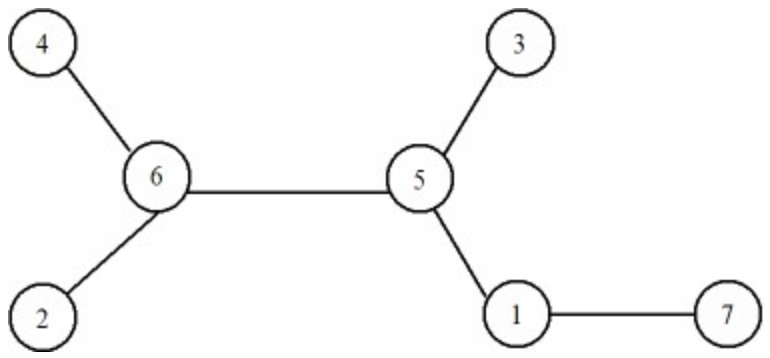시간 제한메모리 제한제출정답맞힌 사람정답 비율
1 초 128 MB1000.000%

## 문제

Let V = {1, 2,…,n} and E ⊂ {{u, v} | 1 ≤ u, v ≤ n}. A tree T = (V, E) is a graph that is connected and has exactly n-1 edges. For example, a tree T1 is shown in the figure below.In T1 we have V = {1, 2, 3, 4, 5, 6, 7} and E = {{4, 6}, {2, 6}, {6, 5}, {3, 5}, {5, 1}, {1, 7}}. Professor Minton has found a way to encrypt a tree. An encrypted tree is a sequence of n-2 numbers from V. For instance, T1 would have sequence <6, 5, 6, 5, 1>. A sequence is broken if some numbers are missing. We use the alphabet x to represent a missing number in the sequence. Given a tree and a broken sequence, write a program to find the missing numbers.

## 입력

The first line is the number t of test cases. The second line is n. The third line is the description of a tree in the form of 2n-2 numbers separated by a blank. The first pair of numbers represents the first edge; the second pair represents the second edge; and so on. The fourth line contains a broken sequence in the form of n-2 numbers, each separated by a blank, where some of these numbers are marked with xʹs to indicate missing.

## 출력

The output contains t lines. The first line contains missing numbers of the first test case in the same order as the inputʹs. The second line contains missing numbers of the second test case in the same order as the inputʹs and so on.

## 예제 입력 1

1
7
4 6 2 6 6 5 3 5 5 1 1 7
6 x x 5 1


## 예제 출력 1

5 6